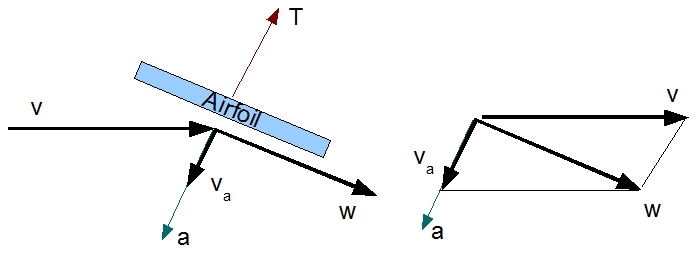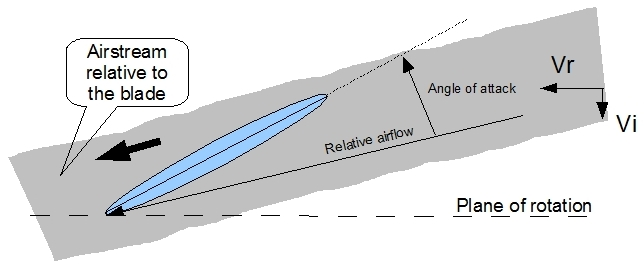# 1 Airfoil

An airfoil can be defined as 'any shape designed to obtain a useful reaction from the air through which it moves'. In helicopters, the rotors are the airfoils which provide lift and propulsion. A helicopter's rotor functions in exactly the same way as an airplane's wing, although the shape of the former differs from that of the latter in that it is much thinner as compared to its length. Another difference is that the airflow through which the rotor moves is mainly generated by rotation. This means that the rotor (functioning as an airfoil) can generate lift even when the aircraft is not moving. It is this mechanism that gives the helicopter its much appreciated V.T.O.L. (Vertical Take Off and Landing) capability.

The airflow in which the rotor blade functions travels towards the blade with speed 'v'. Note that with an airplane, the airflow exists because the plane flies forwards, whereas with the helicopter it is the rotor which travels at a certain speed through the air. The rotor speed is much higher than the airspeed of the helicopter and this is why it can still operate in strong winds. Although there is no principal difference between a rotorblade and an airplane's wing, within this text the context used is always that of a rotating airfoil.The way in which an airfoil generates lift can be explained by more than one principle of physics. In this section, an explanation along the lines of Newton's Laws of Motion is utilised.

As the blades encounter air mass with speed 'v', they accelerate this mass downwards (deflecting), resulting in it (the mass) moving with velocity 'w'. As we know, to accelerate mass requires a working force. This is provided by the (rotating) airfoil moving through the air. But, if there is a force acting from the blade onto the air mass, there must be a  reaction force (equal in magnitude but opposite in direction) acting from the air mass which is working at the blade. It is this reaction force that lifts the blade.

When air is deflected around an airfoil, the direction of T remains orthogonal to it (the airfoil) as long as the deflected airflow follows it (no turbulence or stall). The magnitude of T depends on the amount of air mass involved and the magnitude of acceleration, with the latter being responsible for the vertical velocity component in the airstream.

# 2 Definitions

When studying (rotating) airfoils, we need to know some relevant terms and definitions. These are:

• Chord line: the straight line between the blades' leading and trailing edges.
• Blade angle (or picth angle): angle between a blade's chord line and the plane of rotation.
• Relative airflow (RAF): the Relative Air Flow (RAF) is the airflow with speed and direction, relative to the airfoil.
• Angle of attack: the angle between the RAF (Relative Air Flow) vector and the blade's chord line.
• Inflow angle:. the angle between the RAF (Relative Air Flow) vector and the plane of rotation.
• Induced flow: the airflow which exists solely as a result of the airmass that is forced down by rotoraction.## 2.1 Explanation

The rotor (illustrated here with a symmetrical airfoil) moves at speed Vrotor (due to its angular velocity), and with some pitch (or blade) angle. Now imagine that this angle is zero degrees; there will then be no induced flow. Induced flow only exists when the rotorblade accelerates air downwards, which does not occur when the blade is 100% horizontal. The Relative Airflow (RAF) in this case is also at an angle of zero degrees with the plane of rotation. Seen from the perspective of a rotorblade, the only relevant airflow is the airflow relative to the blade (RAF).

Now imagine that the blade isn't horizontal but is instead at an angle with its plane of rotation: that is, the pitch angle is > 0 zero (e.g. 15 degrees). The blades will now accelerate air downwards, which leads to a vertical speed component in the airstream. This is called the induced flow (induced by the rotorblades). As a result of this, the airstream relative to the rotorblade (the RAF) is no longer parallel to the plane of rotation, but is instead directed slightly downwards (less than the pitch angle and greater than zero degrees). The angle of the RAF changes because of induced flow.The angle between the chord line and the RAF is called the angle of attack. This parameter has a direct relationship to the rotorbladesï¿½ lift and drag forces. The greater the angle of attack, the more lift the blade will produce. An angle of attack of zero results in no lift (assuming a symmetrical airfoil). Increasing the angle of attack has two effects: firstly, more air mass will be accelerated down because of the larger effective area that 'cuts' through the air; secondly, the rate of acceleration also increases as the air is forced down in a steeper angle (greater change of direction, hence more acceleration). Both effects lead to a higher reaction force and, thus, more lift.

Next topic > Lift & Drag

### Helicopter Theory (Paperback)

• This enormous tome provides comprehensive and detailed coverage of every aspect of helicopter theory. The purpose of the book is to set out for the reader the engineering analyses required to enable the successful design of a rotorcraft. All relevant subjects, such as vertical flight, forward flight, performance, rotating systems, rotary wing dynamics, aerodynamics and aero elasticity, and stability and control are covered. They are explained at an in depth engineering level, always accompanied by accurate mathematical analyses. A good knowledge of mathematics and a degree in engineering are necessary, as these topics are dealt with extensively throughout the text.
• 1089 pages.

### Principles of Helicopter Aerodynamics (Cambridge Aerospace Series) (Hardback)

• Modern treatment of the aerodynamic principles of helicopters and rotating-wing vertical lift aircraft
• Uses mathematics throughout the text
• Concepts are derived from basic enginering principals
• 864 pages

# Do you want to comment this topic?

1: (Book) Cyclic and Collective2: (Book) Principles of Helicopter Flight3: Microsoft FSX Steam Edition4: Logitech Extreme 3D Pro Joystick5: Saitek Pro Flight Rudder Pedals